## Block Design

An incidence system (,,,,) in which a setofpoints is partitioned into a familyofsubsets (blocks) in such a way that any two points determineblocks withpoints in each block, and each point is contained indifferent blocks. It is also generally required that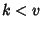, which is where the incomplete'' comes from in the formal term most often encountered for block designs, Balanced Incomplete Block Designs (BIBD). The five parameters are not independent, but satisfy the two relations(1)(2)

A BIBD is therefore commonly written as simply (,,), sinceandare given in terms of,, andby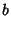(3)(4)

A BIBD is called Symmetric if(or, equivalently,).

Writing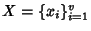and, then the Incidence Matrix of the BIBD is given by the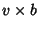Matrixdefined by(5)

This matrix satisfies the equation(6)

where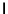is aIdentity Matrix andis amatrix of 1s (Dinitz and Stinson 1992).

Examples of BIBDs are given in the following table.

 Block Design (,,) Affine Plane (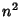,, 1) Fano Plane (7, 3, 1)) Hadamard Design Symmetric (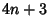,,) Projective Plane Symmetric (,, 1) Steiner Triple System (, 3, 1) Unital (,, 1)

See also Affine Plane, Design, Fano Plane, Hadamard Design, Parallel Class, Projective Plane, Resolution, Resolvable, Steiner Triple System, Symmetric Block Design, Unital

References

Dinitz, J. H. and Stinson, D. R. A Brief Introduction to Design Theory.'' Ch. 1 in Contemporary Design Theory: A Collection of Surveys (Ed. J. H. Dinitz and D. R. Stinson). New York: Wiley, pp. 1-12, 1992.

Ryser, H. J. The-Configuration.'' §8.1 in Combinatorial Mathematics. Buffalo, NY: Math. Assoc. Amer., pp. 96-102, 1963.